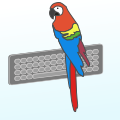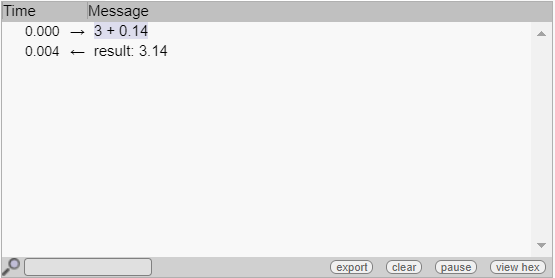Sending values with communication modules

# Sending values with communication modulesYoctopuce serial communication modules such as Yocto-RS485-V2 and Yocto-I2C are capable of autonomously executing small communication protocols, for example to interrogate sensors. We were recently asked whether it was possible to use a value received from a sensor in a message sent later. As is often the case, the answer is: yes, it's now possible...

In the case we were asked to consider, we needed to transmit a humidity measure from an I2C sensor to a gas sensor connected to the same bus, to enable the latter to perform humidity compensation. An I2C readout protocol was used to obtain the required humidity measure, but until now there was no way of sending a parametric message to the second sensor to set the compensation value.

### The new writeVar

If you update your modules' firmware, you can now find a new writeVar command in the drop-down list of commands you can add to your jobs. It behaves like the write command (or writeLine on the Yocto-I2C), but first makes substitutions in the string sent, replacing the following expressions:

• (\$x:INT) sends the value of variable \$x as an integer (decimal)
• (\$x:FLOAT) sends the value of variable \$x as a real number
• (\$x:BYTE) sends the value of variable \$x as a hexadecimal byte (two hexadecimal digits)
• (\$x:WORD) and (\$x:WORDL) send the value of variable \$x as a hexadecimal word (four hexadecimal digits). The L suffix is used to send the value in little-endian format, i.e. with the most significant byte at the end.
• (\$x:DWORD) and (\$x:DWORDL) are used to send the value of variable \$x as a hexadecimal dword (eight hexadecimal digits). The L suffix is used to send the value in little-endian format, i.e. with the most significant byte at the end.
• (\$x:FLOAT32B) and (\$x:FLOAT32L) send the value of the variable \$x in hexadecimal as a 32-bit IEEE 754 floating-point number, respectively with the bytes ordered as big-endian or little-endian.

To ensure that this new functionality would not interfere with our customers' existing protocols, we have chosen to introduce a new command rather than having these expressions recognized in the write command. If you need a line feed after the writeVar, add a writeLine with empty content. Exception: for Yocto-I2C, since sending a message must be atomic, the writeVar does not require a writeLine.

### Short example

For example, if you put the following job into a Yocto-RS232

expect (\$a:FLOAT) + (\$b:FLOAT) compute \$res = \$a + \$b writeVar result: (\$res:FLOAT) writeLine